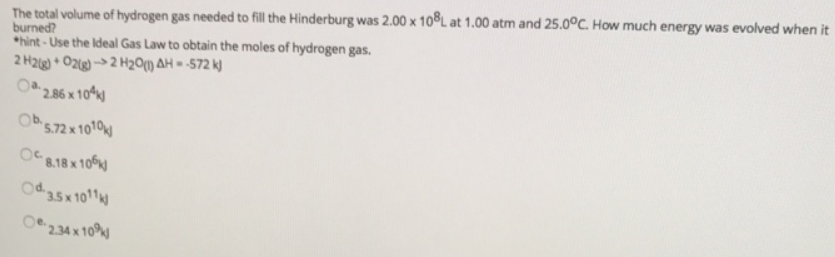# Problem: The total volume of hydrogen gas needed to fill the Hinderburg was 2.00 x 108 L at 1.00 atm and 25.0°C. How much energy was evolved when it burned?Hint-Use the Ideal Gas Law to obtain the moles of hydrogen gas. 2H2 (g) + O2 (g) → 2H2O (l)      ΔH = -572 kJa. 2.86 x 104 kJb. 5.72 x 1010 kJc. 8.18 x 106 kJd. 35 x 1011 kJ e. 2.34 x 109 kJ

###### FREE Expert Solution
80% (436 ratings)###### Problem Details

The total volume of hydrogen gas needed to fill the Hinderburg was 2.00 x 108 L at 1.00 atm and 25.0°C. How much energy was evolved when it burned?

Hint-Use the Ideal Gas Law to obtain the moles of hydrogen gas.

2H2 (g) + O2 (g) → 2H2O (l)      ΔH = -572 kJ

a. 2.86 x 104 kJ

b. 5.72 x 1010 kJ

c. 8.18 x 106 kJ

d. 35 x 1011 kJ

e. 2.34 x 10kJ